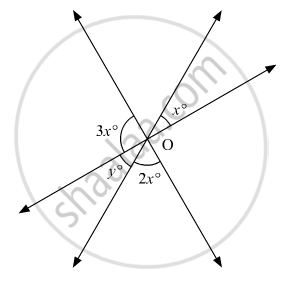# In the Given Figure, the Value Of Y Is - Mathematics

MCQ

In the given figure, the value of y is• 20°

• 30°

• 45°

• 60°

#### Solution

In the given figure, x°and  y°are vertically opposite angles, therefore, these must be equal.That is,

x =y         ...(i)

Also, 2x°, y°and 3x° form a linear pair. Therefore, their sum must be supplementary.

That is,

2x + y + 3x = 180°

From (i) equation, we get:

2x + x +3x = 180°

6x = 180°

x = (180°) /6

x = 30°

From (i) equation again,

y =30°

Concept: Concept of Parallel Lines
Is there an error in this question or solution?

#### APPEARS IN

RD Sharma Mathematics for Class 9
Chapter 10 Lines and Angles
Q 7 | Page 51